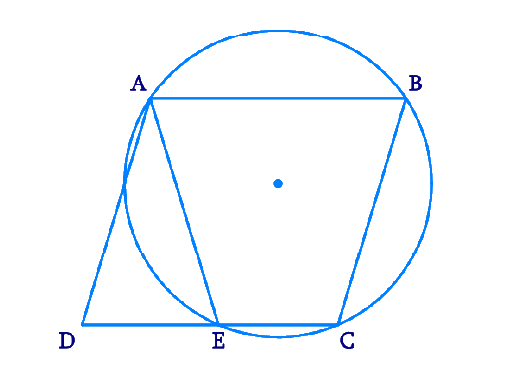In the verge of coronavirus pandemic, we are providing FREE access to our entire Online Curriculum to ensure Learning Doesn't STOP!

# Ex.10.6 Q6 Circles Solution - NCERT Maths Class 9

Go back to  'Ex.10.6'

## Question

\begin {align}{ABCD} \end {align} is a parallelogram. The circle through \begin {align}{A, B} \end {align} and \begin {align}{C} \end {align} intersect \begin {align}{CD} \end {align} (produced if necessary) at \begin {align}{E} \end {align}. Prove that \begin {align}{AE = AD.} \end {align}

Video Solution
Circles
Ex 10.6 | Question 6

## Text Solution

What is known?

\begin {align}{ABCD} \end {align} is a parallelogram.

What is unknown?

Proof that \begin {align}{AE = AD} \end {align}

Reasoning:

• A quadrilateral \begin {align}{ABCD} \end {align} is called cyclic if all the four vertices of it lie on a circle.
• The sum of either pair of opposite angles of a cyclic quadrilateral is $$180^{\circ}.$$
• Opposite angles in a parallelogram are equal.

Steps:We can see that \begin {align}{ABCE} \end {align} is a cyclic quadrilateral.

We know that in a cyclic quadrilateral, the sum of the opposite angles is $$180^{\circ.}$$

\begin{align}\angle {AEC}+\angle {CBA}&=180^{\circ} \\ \angle {AEC} +\angle {AED}&=180^{\circ} (\text { Linear pair })\end{align}

$$\angle {AED}=\angle {CBA} \ldots \text { (1) }$$

We know that in a parallelogram, opposite angles are equal.

\begin {align} \angle {ADE}=\angle{CBA}\quad \dots(2) \end {align}

From ($$1$$) and ($$2$$),

\begin{align} \angle {AED}=\angle{ADE}\\{AD}={AE}\qquad \end{align}

(sides opposite to equal Angles of a triangle are equal).

Video Solution
Circles
Ex 10.6 | Question 6

Learn from the best math teachers and top your exams

• Live one on one classroom and doubt clearing
• Practice worksheets in and after class for conceptual clarity
• Personalized curriculum to keep up with school